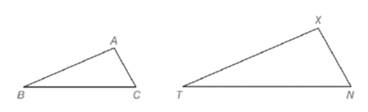Chapter 5.2, Problem 35EElementary Geometry For College St...

7th Edition
Alexander + 2 others
ISBN: 9781337614085

Solutions

Chapter
SectionElementary Geometry For College St...

7th Edition
Alexander + 2 others
ISBN: 9781337614085
Textbook Problem

Given △ A B C , a second triangle ( △ X T N ) is constructed so that ∠ X ≅ ∠ A and ∠ N ≅ ∠ C . a) Is ∠ T congruent to ∠ B ? b) Using intuition (appearance), does it seem that △ X T N is similar to △ A B C ?To determine

a)

Whether T is congruent to B.

Explanation

Approach:

The sum of measure of three angle in a triangle is 180.

Given:

It is given that XA and NC.

Calculation:

In ΔABC,

mA+mB+mC=180mB=180mCmA     (1)

In ΔXTN,

To determine

b)

Whether it seems that ΔABC is similar to ΔXTN.

Still sussing out bartleby?

Check out a sample textbook solution.

See a sample solution

The Solution to Your Study Problems

Bartleby provides explanations to thousands of textbook problems written by our experts, many with advanced degrees!

Get Started

Find more solutions based on key concepts